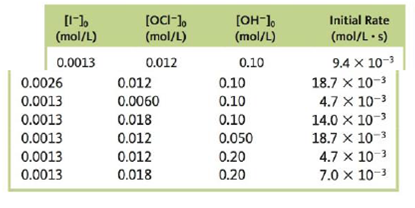Chapter 12, Problem 103CP

Chapter
Section
Textbook Problem

# A study was made of the effect of the hydroxide concentration on the rate of the reaction I − ( a q ) + OCl − ( a q ) → IO − ( a q ) + Cl − ( a q ) The following data were obtained:Determine the rate law and the value of the rate constant for this reaction.

Interpretation Introduction

Interpretation: The data analysis table for the initial concentrations of reactants and initial rate is given. By using these values, the rate law and the value of rate constant is to be determined.

Concept introduction: All chemical reactions follow rate law. This law shows that how the rate of a chemical reaction depends on the concentration of reactants.

Example: Suppose a chemical reaction is as follows:

A+BC+D

According to the rate law, rate of a chemical reaction is expressed by following expression.

Rate=k[A]m[B]n

To determine: The rate law for the given chemical reaction and the value of rate constant.

Explanation

Given

The reaction in which the effect of hydroxide ion concentration on the rate of reaction is analyzed is,

I(aq)+OCl(aq)IO(aq)+Cl(aq)

Data analysis table is as follows:

 [I−]0(mol/L) [OCl−]0(mol/L) [OH−]0(mol/L) Initial rate(mol/L⋅s) 0.0013 0.012 0.10 9.4×10−3 0.0026 0.012 0.10 18.7×10−3 0.0013 0.0060 0.10 4.7×10−3 0.0013 0.018 0.10 14.0×10−3 0.0013 0.012 0.050 18.7×10−3 0.0013 0.012 0.20 4.7×10−3 0.0013 0.018 0.20 7.0×10−3

According to the rate law,

Rate=k[I]m[OCl]n[OH]p

Where,

• k is the rate constant of chemical reaction.
• [I] is the concentration of reactant I .
• [OCl] is the concentration of reactant OCl .
• [OH] is the concentration of reactant OH .
• m is the order of reaction with respect to I .
• n is the order of reaction with respect to OCl .
• p is the order of reaction with respect to OH .

For the first row of table:

Substitute all the values of concentration in the above formula.

9.4×103=k[0.0013]m[0.012]n[0.10]p (1)

For the second row of table:

Substitute all the values of concentration in the above formula.

18.7×103=k[0.0026]m[0.012]n[0.10]p (2)

For the third row of table:

Substitute all the values of concentration in the above formula.

4.7×103=k[0.0013]m[0.0060]n[0.10]p (3)

For the fourth  row of table:

Substitute all the values of concentration in the above formula.

14.0×103=k[0.0013]m[0

### Still sussing out bartleby?

Check out a sample textbook solution.

See a sample solution

#### The Solution to Your Study Problems

Bartleby provides explanations to thousands of textbook problems written by our experts, many with advanced degrees!

Get Started